# 快速排序（JS实现）

### 思想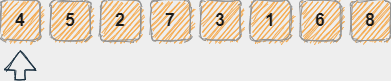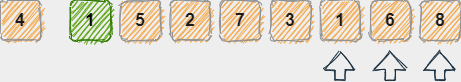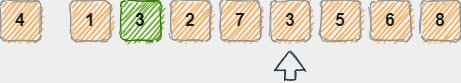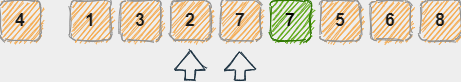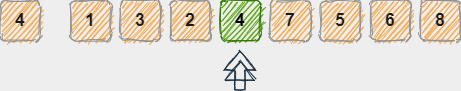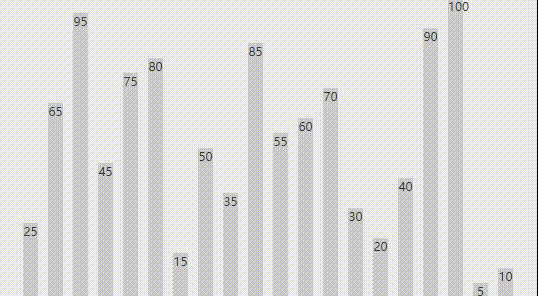### JS实现

const quickSort = (array)=>{
quick(array, 0, array.length - 1)
}

const quick = (array, left, right)=>{
if(left < right){
let index = getIndex(array, left, right);
quick(array, left, index-1)
quick(array, index+1, right)
}
}

const getIndex = (array, leftPoint, rightPoint)=>{
let pivot = array[leftPoint];
while(leftPoint < rightPoint){
while(leftPoint < rightPoint && array[rightPoint] >= pivot) {
rightPoint--;
}
array[leftPoint] = array[rightPoint]
// swap(array, leftPoint, rightPoint) 			//使用swap，则与动态图演示效果完全一致
while(leftPoint < rightPoint && array[leftPoint] <= pivot) {
leftPoint++;
}
array[rightPoint] = array[leftPoint]
// swap(array, leftPoint, rightPoint)
}
array[leftPoint] = pivot
return leftPoint;
}

const swap = (array, index1, index2)=>{
var aux = array[index1];
array.splice(index1, 1, array[index2])
array.splice(index2, 1, aux)
}

const createNonSortedArray = (size)=>{
var array = new Array();
for (var i = size; i > 0; i--) {
//array.push(parseInt(Math.random()*100));
array.push(i*(100/size));
array.sort(function() {
return (0.5-Math.random());
});
}
return array;
}

var arr = createNonSortedArray(20);
quickSort(arr)
console.log(arr)	//[5, 10, 15, 20, 25, 30, 35, 40, 45, 50, 55, 60, 65, 70, 75, 80, 85, 90, 95, 100]


### 时间复杂度

posted @ 2021-05-14 17:02  这个少年有点热丶  阅读(95)  评论(0编辑  收藏  举报